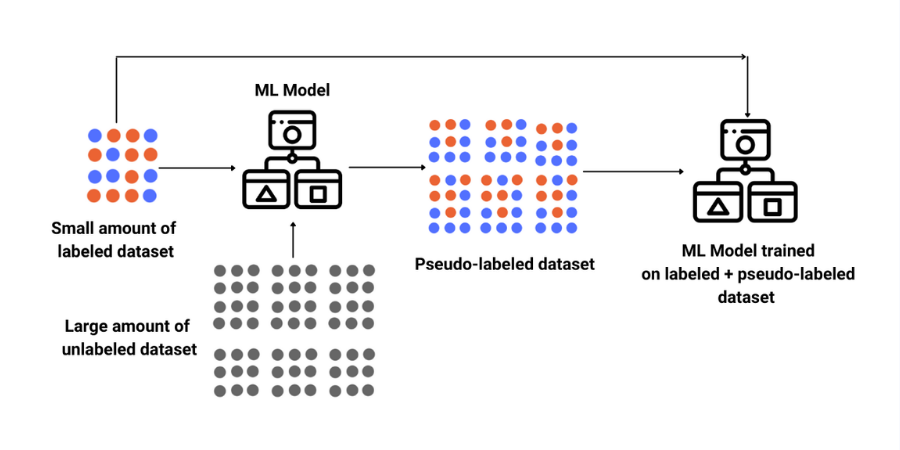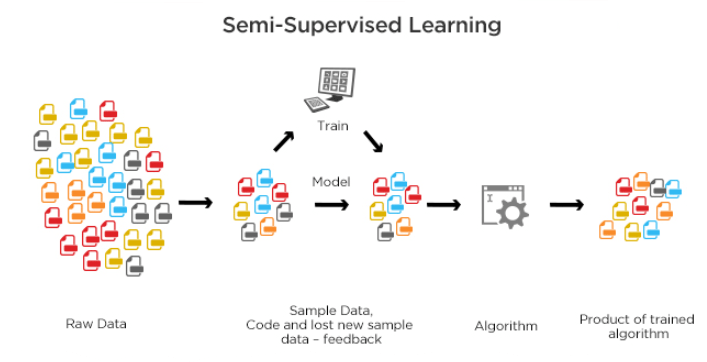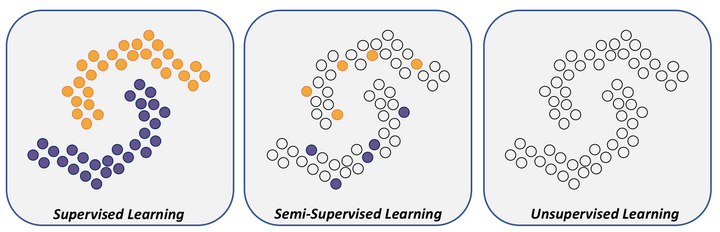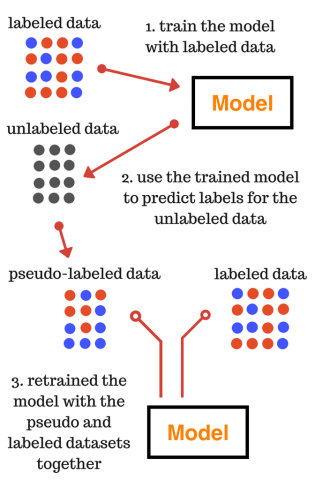# Introduction to Semi-Supervised LearningS.No Lesson Title
1
2
3
4
5

### Introduction

Most of the machine learning algorithms are based on supervised and unsupervised machine learning techniques. The major difference between both techniques is that in the case of supervised learning you have labeled data and in the case of unsupervised learning, the data is not labeled leaving the model to learn on its own to find patterns in data. The idea of allowing the model to learn from its own mistakes leads us to the domain of reinforcement learning, where there is no data and the model learns from the experience and rewards it gets from the learning environment. Then comes semi-supervised learning that is thought to be a fine line between reinforcement learning and unsupervised learning. This looks true at first but when you look closely you'll find striking differences between reinforcement learning and semi-supervised learning. In this article, we'll try to have a look at the different aspects of semi-supervised learning to get a deeper knowledge about this aspect of machine learning.### Why Semi-Supervised Learning?

The most important difference between semi-supervised learning and other machine learning techniques is that it learns using a minimal amount of labeled training data. This also makes it different from reinforcement learning where there is no labeled data involved. But what was the need for semi-supervised learning when we already have supervised learning to learn from labeled data?

The answer lies behind the fact that to train a supervised learning model a large amount of data is needed. This data which is labeled is called annotated data. The process of data annotation is a time-consuming and laborious task when done manually as it requires humans to review each training example before assigning a label to them. This has led to the rise of an entirely new market of labeled data where startups such as LabelBox, ScaleAI, etc. leverage technology to annotate data without any human interference. This looks like a good thing but there's a small catch.

With the increasing popularity of machine learning and demand for labeled data the price of labeled data has increased which has led to machine learning techniques like semi-supervised learning which uses less amount of data to train a ML model. Semi-supervised learning automates the data labeling process when there is a small amount of labeled data by providing labels to unlabeled data with the help of some techniques that take into consideration the distribution of labeled data. The idea of automating labeling is not that straightforward and includes some assumptions on the labeled data at hand. We'll discuss it more in the next section.

### Assumptions and Characteristics of Data used for Semi-Supervised Learning

The classic example of a semi-supervised learning scenario is where the amount labeled data is around fourth or fifth of the total data. Before applying the semi-supervised technique to any kind of data there are a few characteristics that a data scientist should keep in mind:

1. Size of unlabeled portion: Use semi-supervised learning when only a small portion of data is labeled. There isn't an exact ratio to decide when to go for supervised or semi-supervised learning but it can be based on intuition and the type of model that'll be used in both cases. Some supervised learning models like the naïve bayes classifier give good results even for a small dataset.
2. Input-Output Proximity: In supervised learning, the basic approach by many algorithms is to provide a label for an unlabeled input by looking at the labeled data points that are close to it. This means that if the input data points without label lie in a cluster of data points with a particular label then they will be assigned that label. If the labeled data portion has low density then the accuracy of the semi-supervised learning model is affected. Have a look at the following figure to have a better understanding of how the data points for a dataset that can be used for semi-supervised learning look like.
3.4. Simplicity/ Complexity of labeling: If the data is very complex in any aspect then it becomes really difficult to assign labels to data points without a label. This situation can arise when there are a large number of attributes or the number of distinct class labels is high.
• Inductive and Transductive Learning: There are two common approaches to learning when there's a mixed set of a label and unlabeled values. The first one is the method of 'induction'.
• The inductive method of learning is based on the idea that it studies the labeled data and creates a general rule for classification based on reasoning from observations from data. Then all the test cases are included under these general classifications.
• The idea behind transductive learning is to draw reasoning from specific training cases and then apply it to specific test cases. Transductive learning has been supported by the inventor of SVM's Vladimir Vapnik and he gives the reasoning that "Try to get the answer you really need, instead of a general one".

These were a few characteristics about the data or the learning method which should be looked at before applying semi-supervised learning. Let's have a look at few assumptions that are necessary for the structure of data provided:

1. Continuity assumption: Points that are close to each other always have a higher chance of sharing the same label. This gives us geometrically simple decision boundaries. The data is assumed to be continuous in the sense that all the points in the close vicinity of a point are assumed to have the same label.
2. Cluster assumption: It is assumed that data points form clusters with each discrete cluster belonging to one class. This can be considered as a special case of smoothness
3. Manifold assumption: Sometimes the data lies in higher dimensions and it becomes difficult to forms clusters or map the data in those dimensions. In such a case manifold assumption can be used where the data is assumed to be in lower dimensions which makes it easier for our model to learn the data.

These basic assumptions and characteristics make it easy to deal with data used for semi-supervised learning models. In the following section, we'll have a look at the different techniques used for semi-supervised learning.

### Techniques used for Semi-Supervised Learning

There are many techniques or ideas used for semi-supervised learning tasks and here we'll discuss some common ones used:

1. Pseudo Labeling: The idea behind pseudo labeling is simple. Take a training dataset that follows all the assumptions/characteristics and train a model based on this data. Now take some test cases and label them using this model. These labels assigned to the test case might not be accurate and are also known as pseudo labels. Next time is to combine the actual training data and data with pseudo labels to train a model again from scratch.
2.• Generative Learning: The idea behind generative learning is to first estimate the distribution of data points belonging to each class. Semi-supervised learning with a generative approach can be seen as an extension of unsupervised learning (clustering plus some labels) or an extension of supervised learning (classification plus information about probability).
• Generative models assume that distributions take the form p(x|y,Θ) parameterized by the parameter Θ. If these assumptions are incorrect then the unlabeled data can reduce the accuracy of the model which it would have got by only using labeled data. But if assumptions are correct they will necessarily improve the performance.
• The unlabeled data are distributed according to a mixture of individual-class distributions. In order to learn the mixture distribution from the unlabeled data, it must be identifiable, that is, different parameters must yield different summed distributions. Gaussian mixture distributions are identifiable and commonly used for generative models.
3. Another major class of methods attempts to place boundaries in regions with few data points (labeled or unlabeled). One of the most commonly used algorithms is the transductive support vector machine, or TSVM (which, despite its name, may be used for inductive learning as well). Whereas support vector machines for supervised learning seek a decision boundary with a maximal margin over the labeled data, the goal of TSVM is a labeling of the unlabeled data such that the decision boundary has maximal margin over all of the data.

There are many more techniques that can be used for semi-supervised learning. We have discussed the most commonly used ones to give a basic idea about how semi-supervised learning algorithms work.

### Conclusion

Semi-supervised learning comprises techniques whose knowledge can help our models in doing their job with a low amount of labeled data which leads to cost reduction and improvement in training time. One should be careful before applying semi-supervised learning as sometimes supervised models might end up giving better accuracy for the same amount of training data. Happy learning!

Send Message
Top